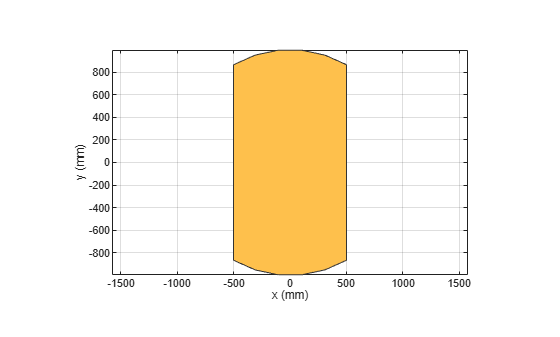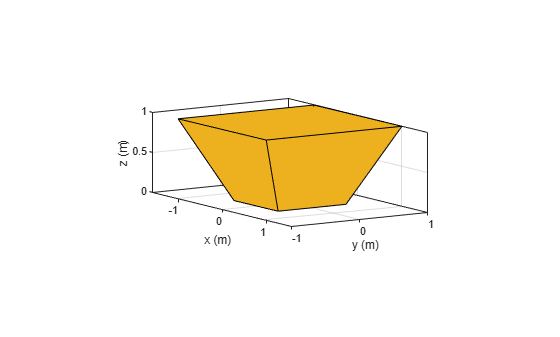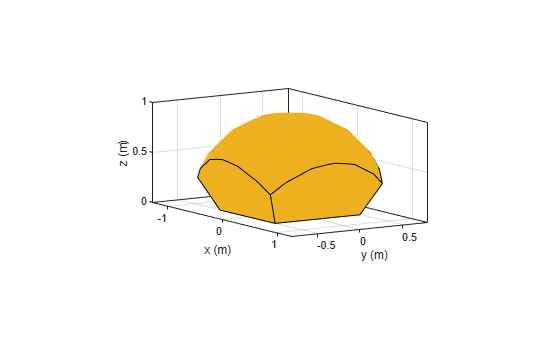# and

Boolean intersection operation on two shapes

## Syntax

``and(shape1,shape2)``
``c = and(shape1,shape2)``

## Description

example

````and(shape1,shape2)` plots a shape generated by intersecting the`shape1` and `shape2`. Alternatively, you can also use the `'&'` operator to intersect the shapes (```shape1 & shape2```).```

example

````c = and(shape1,shape2)` generates the shape obtained by intersecting `shape1` and `shape2` and returns a polygon object for resultant 2-D shape or a custom 3-D object for resultant 3-D shape. Alternatively, you can also use the `'&'` operator to intersect the shapes (```c = shape1 & shape2```).```

## Examples

collapse all

```Create a rectangular and circular shape and intersect them. ```
```r = antenna.Rectangle; c = antenna.Circle; r&c;```This example shows how to create a custom shape using a 3-D trapezoid and sphere.

Create and view a 3-D trapezoid.

```a = shape.Custom3D(Color=[0.9290 0.6940 0.1250]); show(a)```Create and view a sphere.

```b = shape.Sphere(Color=[0.9290 0.6940 0.1250]); show(b)```Create and view the shape formed by the intersection of these two shapes.

```c = and(a,b); show(c)```## Input Arguments

collapse all

2-D and 3-D geometric shapes created using shape objects in Custom 2-D and 3-D Antenna.

Example: `antenna.Rectangle,antenna.Circle`

Example: `shape.Box,shape.Sphere`

## Output Arguments

collapse all

Shape obtained after intersecting two 2-D or 3-D shapes.

Example: `antenna.Polygon`

Example: `shape.Polygon`

Example: `shape.Custom3D`

## Version History

Introduced in R2017a